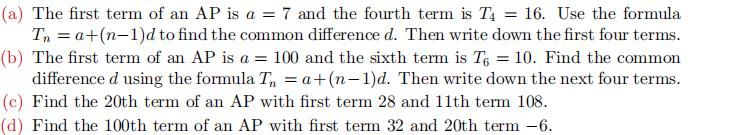### Still have math questions?

Algebra
Question(a) The first term of an AP is $$a = 7$$ and the fourth term is $$T _ { 4 } = 16$$ . Use the formula

$$T _ { n } = a + ( n - 1 ) d$$ to find the common difference $$d$$ . Then write down the first four terms. (b) The first term of an AP is $$a = 100$$ and the sixth term is $$T _ { 6 } = 10$$ . Find the common difference $$d$$ using the formula $$T _ { n } = a + ( n - 1 ) d$$ . Then write down the next four terms. (c) Find the $$20$$ th term of an AP with first term $$28$$ and $$11$$ th term $$108$$ . (d) Find the $$100$$ th term of an AP with first term $$32$$ and $$20$$ th term $$- 6$$ .

15(a) $$d = 3 ; 7,10,13,16 \quad$$ (b) $$d = - 18 ; 82,64,46$$
$$28 \quad$$ (c) $$d = 8 ; T _ { 20 } = 180 \quad$$ (d) $$d = - 2 ; T _ { 100 } = - 166$$